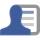# Over-unity Physics. Electricity

 Price 70 USD Оформить заказ

The second part of the book Over-unity Physics - Electricity, includes information about physical processes occurring during electrical phenomena. The essence of electric current and electric flow as basic phenomena is described, on the basis of which the phenomenon of surplus energy is possible. The principles of obtaining free energy are explained.

Content:

Chapter 5. Electric current and potential.
5.1. Atomic electric current in the conductor.
5.1.1. The phenomenon of electrical resistance.
5.1.2. Atomic electric current in electrolytes.
5.2. Electric atomic potential.
5.2.1. The difference in electrical potentials.
5.3. The relationship of atomic current and potential.
5.4. The potential on semiconductors and in dielectrics.
5.5. Electric current in semiconductors.

Chapter 6. Electrostatics. The flow of charges.
6.1. The emergence of electric charges.
6.2. Electrostatic charge on the bodies.
6.3. Electrostatic charge and electric potential.
6.4. Options for creating an electrostatic charge.
6.5. Separation of electric charges.
6.6. Anniygilation of electric charges.
6.7. Separation of electric charges.
6.8. Electric charges in a magnetic field.
6.9. Electric domains.
6.10. Electrostatic engines. Chapter

7. Free energy.
7.1.1. How to increase the circular speed of the electron?
7.3. Delta generation.
7.3.1. Mercury generator.
7.3.2. Generator on the discharge.
7.3.3. Diodes instead of a discharge.
7.3.4. Generator on orthogonal magnetic fields.
7.3.5. Acoustic generators.Аноним От Земли отвязан качер? Нагрузка может быть только активная?Админ Без земли будет накапливаться статика. Нагрузка активная.mi Какой коэффициент эффективности входной и выходной мощности этого устройства?Админ Несколько единиц. Точно никто не измерял.